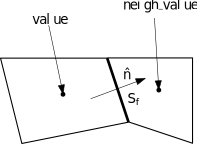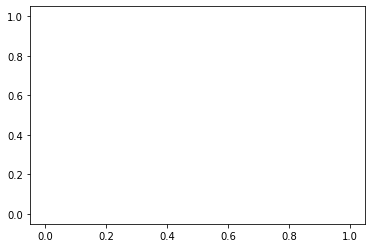# Implementing a solver for the advection equation¶

We’re going to write all the necessary objects to solve the following equation:That, compared to our generalized model:Gives,So our problem needs just to provide.## State¶

from josie.state import State
from josie.fields import Fields

phi = 0



## Problem¶

We just need to implement theoperator

from josie.problem import Problem

Problem??

from josie.mesh.cellset import CellSet, MeshCellSet

V = np.array([1, 0])

DIMENSIONALITY = 2 ## 2D

def B(self, cells: CellSet):
values: AdvectionState = cells.values
nx, ny, num_fields = values.shape
fields = values.fields

B = np.zeros((nx, ny, num_fields, num_fields, self.DIMENSIONALITY))

B[..., fields.phi, fields.phi, : ] = self.V

return B


## Scheme¶We just need to implement thefrom josie.scheme.nonconservative import NonConservativeScheme

NonConservativeScheme??


class Upwind(NonConservativeScheme):
def G(self, cells: MeshCellSet, neighs: CellSet):
V = self.problem.V

# Get the normal velocities (that are equal everywhere) per each cell
Vn = np.einsum("k,...kl->...l", V, neighs.normals[..., np.newaxis])

values_face = np.zeros_like(cells.values)

# We use the cell value where Vn > 0
np.copyto(values_face, cells.values, where=(Vn>0))

# We use the neighbour value otherwise
np.copyto(values_face, neighs.values, where=(Vn<=0))

# Multiply by the normal
valuesn_face = np.einsum("...i,...j->...ij", values_face, neighs.normals)

# Multiply by the surface
G = valuesn_face * neighs.surfaces[..., np.newaxis, np.newaxis]

return G


## Mesh¶

We generated a 1D mesh

from josie.boundary import Line

left = Line([0, 0], [0, 1])
bottom = Line([0, 0], [1, 0])
right = Line([1, 0], [1, 1])
top = Line([0, 1], [1, 1])


We apply periodic boundary condition along the x-axis (no BC on y-axis since it’s a 1D simulation)

from josie.bc import make_periodic, Direction

left, right = make_periodic(left, right, Direction.X)
top.bc = None
bottom.bc = None

print(left, right, top, bottom)

<josie.boundary.boundary.Line object at 0x7f30ec5cf3a0> <josie.boundary.boundary.Line object at 0x7f30ec5cf340> <josie.boundary.boundary.Line object at 0x7f30ec5cf580> <josie.boundary.boundary.Line object at 0x7f30ec5cf460>

from josie.mesh import Mesh
from josie.mesh.cell import SimpleCell
mesh = Mesh(left, bottom, right, top, SimpleCell)
mesh.interpolate(300, 1)
mesh.generate()


## Solver¶

Let’s assemble our scheme, that still needs the time scheme, and a CFL method

from josie.general.schemes.time import ExplicitEuler

def CFL(
self,
cells: MeshCellSet,
CFL_value: float,
) -> float:

U_abs = np.linalg.norm(self.problem.V)
dx = np.min(cells.surfaces)

return CFL_value * dx / U_abs


from josie.solver import Solver

solver = Solver(mesh, AdvectionState, scheme)


We need now to define an initialization function to initialize the domain

def init_fun(cells: MeshCellSet):
xc = cells.centroids[..., 0]

xc_r = np.where(xc >= 0.5)
xc_l = np.where(xc < 0.5)

cells.values[xc_r, xc_r, ...] = 1
cells.values[xc_l, xc_l, ...] = 0


### Run the simulation¶

Let’s initialize the solver state

solver.init(init_fun)

# Plotting stuff
import matplotlib.pyplot as plt
import copy

from matplotlib.animation import ArtistAnimation
solution = []

t = 0
final_time = 1
CFL = 0.5


Let’s iterate in time choosing a time-based writing strategy (save every) to memory

from josie.io.write.strategy import TimeStrategy
from josie.io.write.writer import MemoryWriter

strategy = TimeStrategy(dt_save=0.01)
writer = MemoryWriter(strategy, solver, final_time, CFL)
writer.solve()

fig, ax = plt.subplots()
ims = []

for solution in writer.data:
cells = solution.mesh.cells
x = cells.centroids[..., 0]
x = x.reshape(x.size)
phi = cells.values[..., AdvectionFields.phi]
(im,) = ax.plot(x, phi, 'ko-')
ims.append([im])

ani = ArtistAnimation(fig, ims, interval=50)